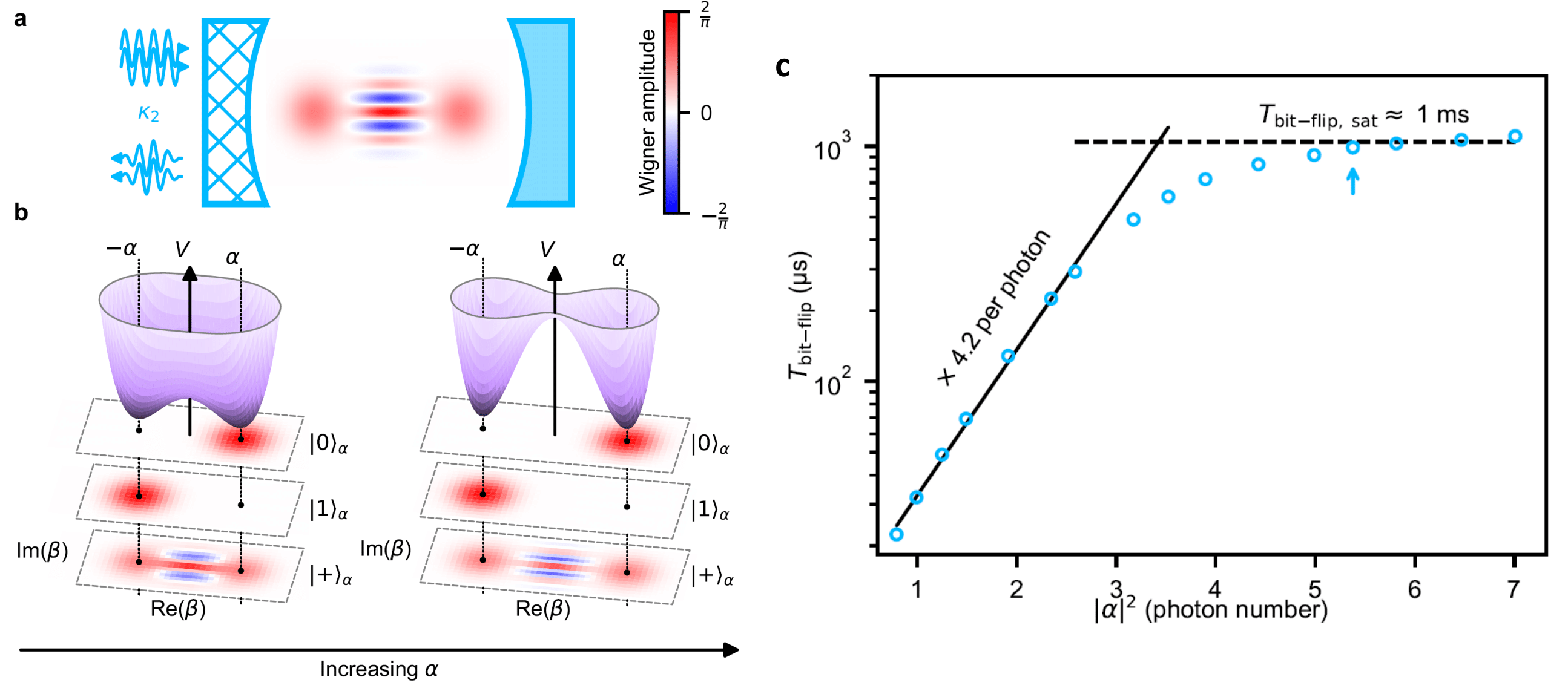A quantum system interacts with its environment—if ever so slightly—no matter how much care is put into isolating it. Therefore, quantum bits undergo errors, putting dauntingly difficult constraints on the hardware suitable for quantum computation. New strategies are emerging to circumvent this problem by encoding a quantum bit non-locally across the phase space of a physical system. Because most sources of decoherence result from local fluctuations, the foundational promise is to exponentially suppress errors by increasing a measure of this non-locality. Prominent examples are topological quantum bits, which delocalize information over real space and where spatial extent measures non-locality.

In a paper published in Nature Physics, an LPENS/Mines/INRIA team follow a new route. They encode a quantum bit in the field quadrature space of a superconducting resonator endowed with a special mechanism that dissipates photons in pairs. This process pins down two computational states to separate locations in phase space. By increasing this separation, they measure an exponential decrease of the bit-flip rate while only linearly increasing the phase-flip rate. Because bit-flips are autonomously corrected, only phase-flips remain to be corrected via a one-dimensional quantum error correction code. This exponential scaling demonstrates that resonators with nonlinear dissipation are promising building blocks for quantum computation with drastically reduced hardware overhead.Figure : The cat-qubit. (a) Quantum information is encoded in a resonator (blue mirrors) coupled to its environment through a special apparatus (hatched mirror) where pairs of photons are exchanged (double arrows). (b) This dynamics is illustrated by a pseudo-potential V (purple) defined over the resonator quadrature phase space. The cat-qubit states lie in the minima of V and are separated in phase space as shown by their Wigner representations (stacked color plots). Bit-flip errors are exponentially suppressed by increasing this separation. Crucially, this pseudo-potential does not alter quantum superpositions such as a Schrödinger cat state. (c) Measured bit-flip time as a function of cat size. We observe an exponential increase and reach a bit-flip time 300 times longer than the intrinsic cavity lifetime.

More :
https://doi.org/10.1038/s41567-020-0824-x

Author affiliation :
Laboratoire de Physique de L’Ecole normale supérieure (LPENS, ENS Paris/CNRS/Sorbonne Université/Université de Paris)

Corresponding author : Zaki Leghtas

Communication contact : Communication team